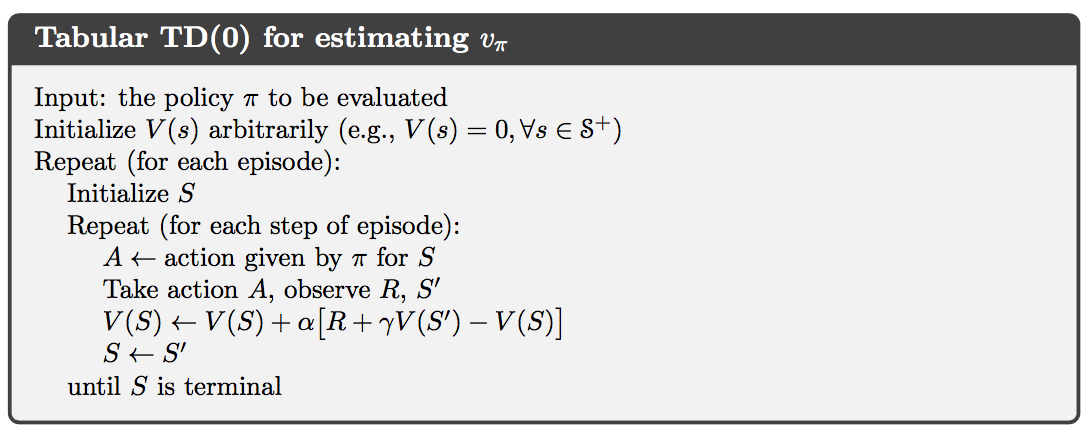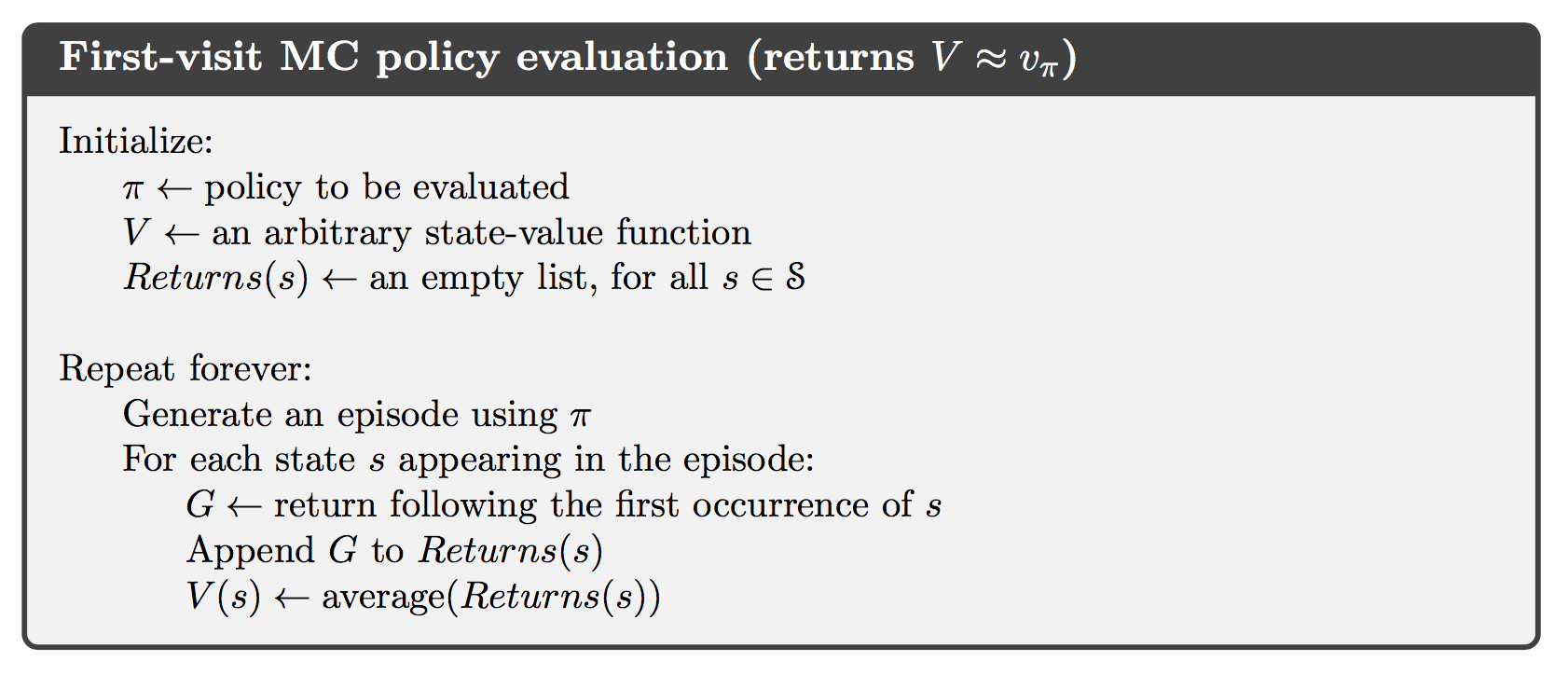# [Notes on Mathematics for ESL] Chapter 4: Linear Methods for Classification

### 4.3 Linear Discriminant Analysis

#### Derivation of Equation (4.9)

For that each class’s density follows multivariate Gaussian

Take the logarithm of $f_k(x)$, we get

where $c = -\log [(2\pi)^{p/2}\lvert\Sigma\rvert^{1/2}]$ and $\mu_k^T\Sigma^{-1}x=x^T\Sigma^{-1}\mu_k$. Following the above formula, we can derive Equation (4.9) easily

# [Notes on Mathematics for ESL] Chapter 3: Linear Regression Models and Least Squares

### 3.2 Linear Regression Models and Least Squares

#### Derivation of Equation (3.8)

The least squares estimate of $\beta$ is given by the book’s Equation (3.6)

From the previous post, we know that $\mathrm{E}(\mathbf{y})=X\beta$. As a result, we obtain

Then, we get

The variance of $\hat \beta$ is computed as

If we assume that the entries of $\mathbf{y}$ are uncorrelated and all have the same variance of $\sigma^2$, then $\mathrm{Var}(\varepsilon)=\sigma^2I_N$ and the above equation becomes

This completes the derivation of Equation (3.8).

# [Notes on Mathematics for ESL] Chapter 2: Overview of Supervised Learning

### 2.4 Statistical Decision Theory

#### Derivation of Equation (2.16)

The expected predicted error (EPE) under the squared error loss:

Taking derivatives with respect to $\beta$:

In order to minimize the EFE, we make derivatives equal zero which gives Equation (2.16):

Note: $x^T\beta$ is a scalar, and $\beta$ is a constant.

# 统计释疑(1)：什么是p值

2014 1000 350 30 8.57%
2015 1000 650 10 1.54%
2016 1000 200 10 5.00%

# Notes on Reinforcement Learning (4): Temporal-Difference Learning

Temporal-difference (TD) learning is a combination of Monte Carlo ideas and dynamic programming (DP) ideas.

### TD Prediction

Both TD and Monte Carlo methods use experience to solve the prediction problem. Given some experience following a policy $\pi$, both methods update their estimate $v$ of $v_\pi$ for the nonterminal states $S_t$ occurring in that experience. Whereas Monte Carlo methods must wait until the end of the episode to determine the increment to $V(S_t)$ (only then is $G_t$ known), TD methods need wait only until the next time step. The simplest TD method, known as TD(0), is

TD methods combine the sampling of Monte Carlo with the bootstrapping of DP. As we shall see, with care and imagination this can take us a long way toward obtaining the advantages of both Monte Carlo and DP methods.# Notes on Reinforcement Learning (3): Monte Carlo Methods

In particular, suppose we wish to estimate $v_\pi(s)$, the value of a state $s$ under policy $\pi$, given a set of episodes obtained by following $\pi$ and passing through $s$. Each occurrence of state $s$ in an episode is called a visit to $s$. The first-visit MC method estimates $v_\pi(s)$ as the average of the returns following from first visits to $s$, whereas every-visit MC method averages the returns following all visits to $s$.